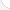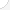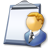MathematiciansDescription: Mathematicians work in two areas: theory and applications. Theoretical mathematicians look for relationships between new math principles and old ones. This can help in science and engineering. Applied mathematicians use math to solve problems in business, government, and everyday life. Many mathematicians teach at colleges and universities.Source: Bureau of Labor Statistics, U.S. Department of Labor, Occupational Outlook Handbook, 2008-09 Edition at http://www.bls.gov/OCO/Complete Job Profile: http://www.bls.gov/oco/ocos043.htmSalary: \$50,001 or more per yearComments:

There are 71 math topics Mathematicians need to know.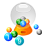Basic Math / Algebra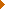FractionsDecimalsRatio and ProportionPercentCustomary MeasurementMetric MeasurementMeasurement ConversionBasic ProbabilityBasic StatisticsStatistical GraphingPowers and RootsOther Number BasesNegative NumbersScientific NotationBasic Problem Solving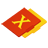First-Year AlgebraUsing FormulasLinear EquationsLinear InequalitiesOperations with PolynomialsFactoring PolynomialsRational ExpressionsCoordinate Graphing 2DLinear SystemsRadicalsQuadratic EquationsAlgebraic Representation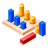GeometryBasic TerminologyAngle MeasurementCongruent TrianglesTriangle InequalitiesParallel LinesQuadrilateralsSimilarityGeometric MeanPythagorean TheoremRight Triangle TrigonometryCirclesConstructionsAreaVolumeTransformationsMake/Use 3D Drawings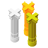Second-Year Algebra / TrigonometryFunctionsVariationImaginary NumbersPolynomial EquationsLogarithmsSequences and SeriesMatricesCoordinate Graphing 3DAdvanced ProbabilityAdvanced StatisticsConic SectionsNon-Linear SystemsTrigonometric/Circular FunctionsGraphs of Trigonometric FunctionsTrigonometric IdentitiesTrigonometric Equations/InversesOblique TrianglesPolar Coordinates/GraphsVectors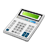Other TopicsCalculus and Higher MathBasic Calculator UseScientific Calculator UseComputer UseComputer ProgrammingGroup Problem SolvingMental MathInductive/Deductive ReasoningMath CommunicationsMathematical Modeling